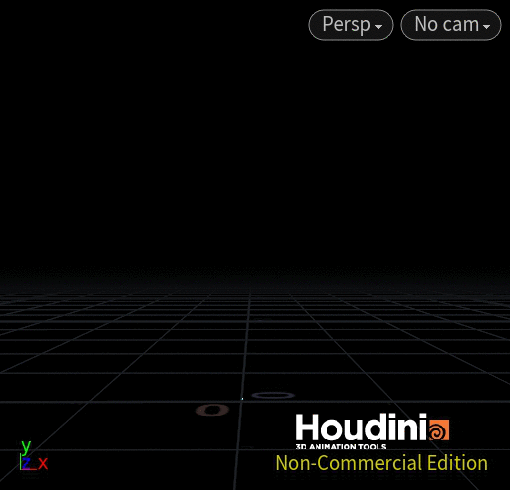Recommended Posts

HI Experts,

Im trying to get some fireworks forming to smiley emoji.. Similar to the fireworks in Tron Movie. what would be great solution to get this type of effect.

sample hip file would be helpful.

Thanks a lot in advance.

CM

Share on other sites

people would be more keen to share an example file with you if you uploaded one as well showing that you have tried to recreate this FX on your own.

•2

Share on other sites

Here's an idea : )•4

Share on other sites

@jkunz07 great file! very interesting how you got it to work with vex. I understand the random input part but I am a little confused on this part in the point expression :

floor(random(i@id)*npoints(which_input))) / vector(2.);

what exactly is going on here ? why do we need to no the npoints? Also, what exactly is vector(2.); doing ?

thanks for you time !

Share on other sites

Of course.

The reason for the npoints()

the random() just gives us a number between 0-1, but we want to use a random point of the reference geometry (the face symbol text)

we multiply the 0-1 number by the number of points (npoints() ) of the reference geometry so that then have a random number which corresponds to a point on that geometry.  If we have a guide shape with 32 points, we want to get a random number between 0 and 32, not simply 0 and 1.

The reason for the vector()

The point function itself can be used to retrieve lot's of different data types, not only vectors like position (P).  You could use it to find the pscale of a point which would be a float, or the id of a point which would be an int.

If we don't explicitly tell the point function which data type it's finding, it will try to do it's best to guess.  If we divide by a float after the point function, the VEX compiler might guess we are pulling float information using the point function and we'd miss getting the y and z components of the position.

Specifying vector(2.) is the same as doing {2,2,2}.  It just forces that to be a vector instead of a float.  I usually try to be overly careful when specifying things, this is why I leave the decimal after some numbers, so I can be sure it's interpreted by the compiler as a float data type.  In some languages like Python or HLSL if you just type "2" instead of "2." it will assume that's an int data type.  That could give you errors, or unexpected results.

Let me know if any of that doesn't make sense or you want to know more.

Create an account or sign in to comment

You need to be a member in order to leave a comment

Create an account

Sign up for a new account in our community. It's easy!

Register a new account